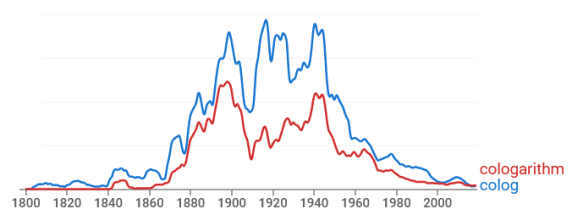# Cologarithms and Entropy

The term “cologarithm” was once commonly used but now has faded from memory. Here’s a plot of the frequency of the terms cololgarithm and colog from Google’s Ngram Viewer.The cologarithm base b is the logarithm base 1/b, or equivalently, the negative of the logarithm base b.

cologb x = log1/b x = -logb x

I suppose people spoke of cologarithms more often when they did calculations with logarithm tables.

## Entropy

There’s one place where I would be tempted to use the colog notation, and that’s when speaking of Shannon entropy.

The Shannon entropy of a random variable with N possible values, each with probability pi, is defined to beAt first glace this looks wrong, as if entropy is negative. But you’re taking the logs of numbers less than 1, so the logs are negative, and the negative sign outside the sum makes everything positive.

If we write the same definition in terms of cologarithms, we havewhich looks better, except it contains the unfamiliar colog.

## Bits, nats, and dits

These days entropy is almost always measured in units of bits, i.e. using b = 2 in the definition above. This wasn’t always the case.

When logs are taken base e, the result is in units of nats. And when the logs are taken base 10, the result is in units of dits.

So binary logs give bits, natural logs give nats, and decimal logs give dits.

Bits are sometimes called “shannons” and dits were sometimes called “bans” or “hartleys.” The codebreakers at Bletchley Park during WWII used bans.

The unit “hartley” is named after Ralph Hartley. Hartley published a paper in 1928 defining what we now call Shannon entropy using logarithms base 10. Shannon cites Hartley in the opening paragraph of his famous paper A Mathematical Theory of Communication.